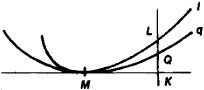# Osculation

Also found in: Dictionary, Thesaurus, Medical, Wikipedia.
The following article is from The Great Soviet Encyclopedia (1979). It might be outdated or ideologically biased.

## Osculation

The osculation of a curve q with a curve l at a given point M is a geometric concept meaning that q at M has contact with l of maximum order by comparison with the other curves in some preassigned family of curves {q} containing q.Figure 1

The order of contact of q and l is n if the length of the segment QL is an infinitesimal of order n + 1 relative to the length of the segment MK (see Figure 1). Here, QL is perpendicular, when extended, to the common tangent of q and l at M. Thus, with respect to the curves in {q}, the curve that is closest to l—that is, the curve for which the length of QL is an infinitesimal of maximum order—is the osculating curve of l at M. For example, the osculating circle of l at M is the circle whose order of contact with l is greater than that of any other circle.

The osculation of a surface q belonging to a given family of surfaces {q} with some curve / or with some surface at the point M of the curve or surface can be defined in much the same way. The order of contact here is defined in a manner similar to the above. We need only replace the tangent line MK shown in Figure 1 by the tangent plane of q at M.

### REFERENCES

La Vallée Poussin, C.-J. de. Kurs analiza beskonechno malykh, vol. 2. Leningrad-Moscow, 1933. (Translated from French.)
Il’in, V. A., and E. G. Pozniak. Osnovy matematicheskogo analiza, 3rd ed., part 1. Moscow, 1971.